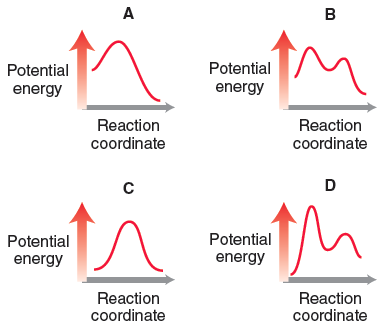# Problem: Consider the following four energy diagrams:Compare diagrams A and C. Which has a negative ΔG?

###### FREE Expert Solution
88% (158 ratings)
###### Problem Details

Consider the following four energy diagrams:

Compare diagrams A and C. Which has a negative ΔG?Frequently Asked Questions

What scientific concept do you need to know in order to solve this problem?

Our tutors have indicated that to solve this problem you will need to apply the Gibbs Free Energy concept. You can view video lessons to learn Gibbs Free Energy. Or if you need more Gibbs Free Energy practice, you can also practice Gibbs Free Energy practice problems.

What is the difficulty of this problem?

Our tutors rated the difficulty ofConsider the following four energy diagrams:Compare diagrams...as medium difficulty.

What textbook is this problem found in?

Our data indicates that this problem or a close variation was asked in Organic Chemistry - Klein 1st Edition. You can also practice Organic Chemistry - Klein 1st Edition practice problems.